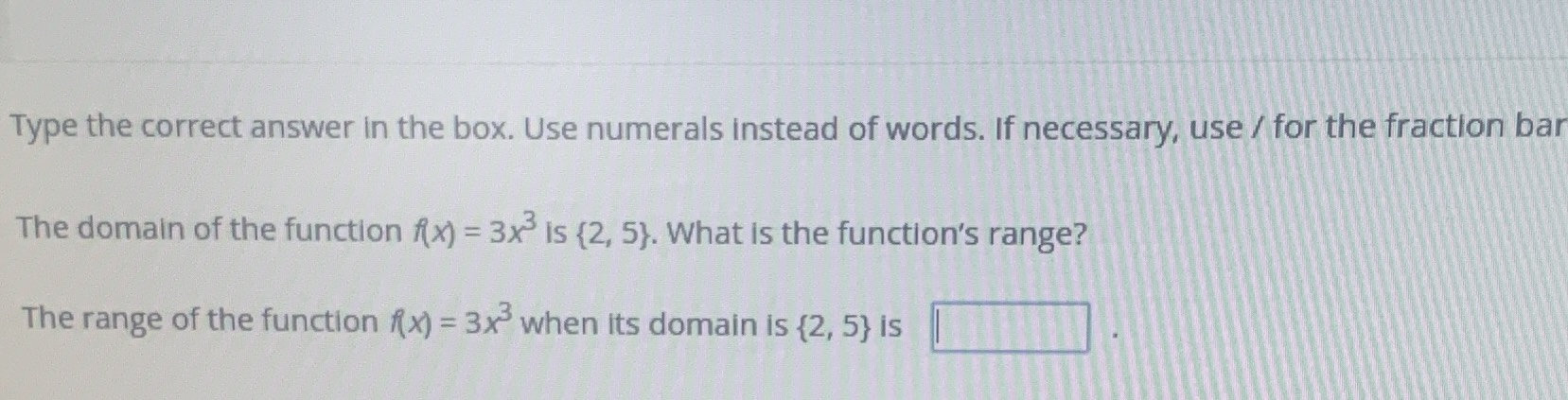### Still have math questions?

Algebra
QuestionType the correct answer in the box. Use numerals instead of words. If necessary, use / for the fraction bar.

The domain of the function $$f ( x ) = 3 x ^ { 3 }$$ is $$\{ 2,5 \}$$ . What is the function's range?

The range of the function $$f ( x ) = 3 x ^ { 3 }$$ when its domain is $$\{ 2,5 \}$$ is $$\square$$